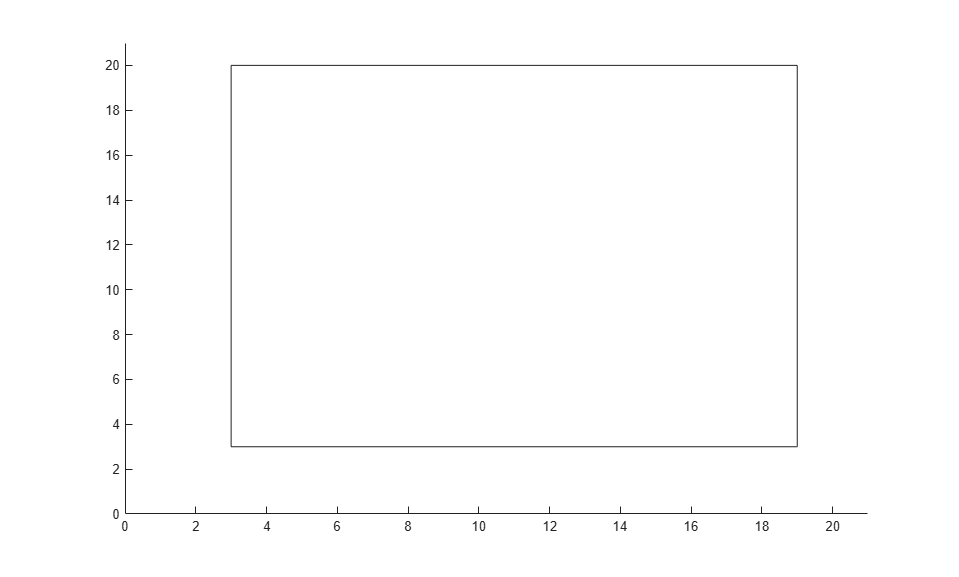Custom Output Function for Genetic Algorithm

This example shows the use of a custom output function in the genetic algorithm solver ga. The custom output function performs the following tasks:

• Plot the range of the first two components of the population as a rectangle. The left and lower sides of the rectangle are at the minima of x(1) and x(2) respectively, and the right and upper sides are at the respective maxima.

• Halt the iterations when the best function value drops below 0.1 (the minimum value of the objective function is 0).

• Record the entire population in a variable named gapopulationhistory in your MATLAB® workspace every 10 generations.

• Modify the initial crossover fraction to the custom value 0.2, and then update it back to the default 0.8 after 25 generations. The initial setting of 0.2 causes the first several iterations to search primarily at random via mutation. The later setting of 0.8 causes the following iterations to search primarily via combinations of existing population members.

Objective Function

The objective function is for four-dimensional x whose first two components are integer-valued.

function f = gaintobj(x)
f = rastriginsfcn([x(1)-6 x(2)-13]);
f = f + rastriginsfcn([x(3)-3*pi x(4)-5*pi]);

Output Function

The custom output function sets up the plot during initialization, and maintains the plot during iterations. The output function also pauses the iterations for 0.1s so you can see the plot as it develops.

function [state,options,optchanged] = gaoutfun(options,state,flag)
persistent h1 history r
optchanged = false;
switch flag
case 'init'
h1 = figure;
ax = gca;
ax.XLim = [0 21];
ax.YLim = [0 21];
l1 = min(state.Population(:,1));
m1 = max(state.Population(:,1));
l2 = min(state.Population(:,2));
m2 = max(state.Population(:,2));
r = rectangle(ax,'Position',[l1 l2 m1-l1 m2-l2]);
history(:,:,1) = state.Population;
assignin('base','gapopulationhistory',history);
case 'iter'
% Update the history every 10 generations.
if rem(state.Generation,10) == 0
ss = size(history,3);
history(:,:,ss+1) = state.Population;
assignin('base','gapopulationhistory',history);
end
% Find the best objective function, and stop if it is low.
ibest = state.Best(end);
ibest = find(state.Score == ibest,1,'last');
bestx = state.Population(ibest,:);
bestf = gaintobj(bestx);
if bestf <= 0.1
state.StopFlag = 'y';
disp('Got below 0.1')
end
% Update the plot.
figure(h1)
l1 = min(state.Population(:,1));
m1 = max(state.Population(:,1));
l2 = min(state.Population(:,2));
m2 = max(state.Population(:,2));
r.Position = [l1 l2 m1-l1 m2-l2];
pause(0.1)
% Update the fraction of mutation and crossover after 25 generations.
if state.Generation == 25
options.CrossoverFraction = 0.8;
optchanged = true;
end
case 'done'
% Include the final population in the history.
ss = size(history,3);
history(:,:,ss+1) = state.Population;
assignin('base','gapopulationhistory',history);
end

Problem Setup and Solution

Set the lower and upper bounds.

lb = [1 1 -30 -30];
ub = [20 20 70 70];

Set the integer variables and number of variables.

intcon = [1 2];
nvar = 4;

Set options to call the custom output function, and to initially have little crossover.

options = optimoptions('ga','OutputFcn',@gaoutfun,'CrossoverFraction',0.2);

For reproducibility, set the random number generator.

rng default

Set the objective function and call the solver.

fun = @gaintobj;
[x,fval] = ga(fun,nvar,[],[],[],[],lb,ub,[],intcon,options)
Got below 0.1
Optimization terminated: y

x =

6.0000   13.0000    9.4201   15.7052

fval =

0.0059The output function halted the solver.

View the size of the recorded history.

disp(size(gapopulationhistory))
40     4     6

There are six records of the 40-by-4 population matrix (40 individuals, each a 4-element row vector).

Global Optimization Toolbox DocumentationGet trial now